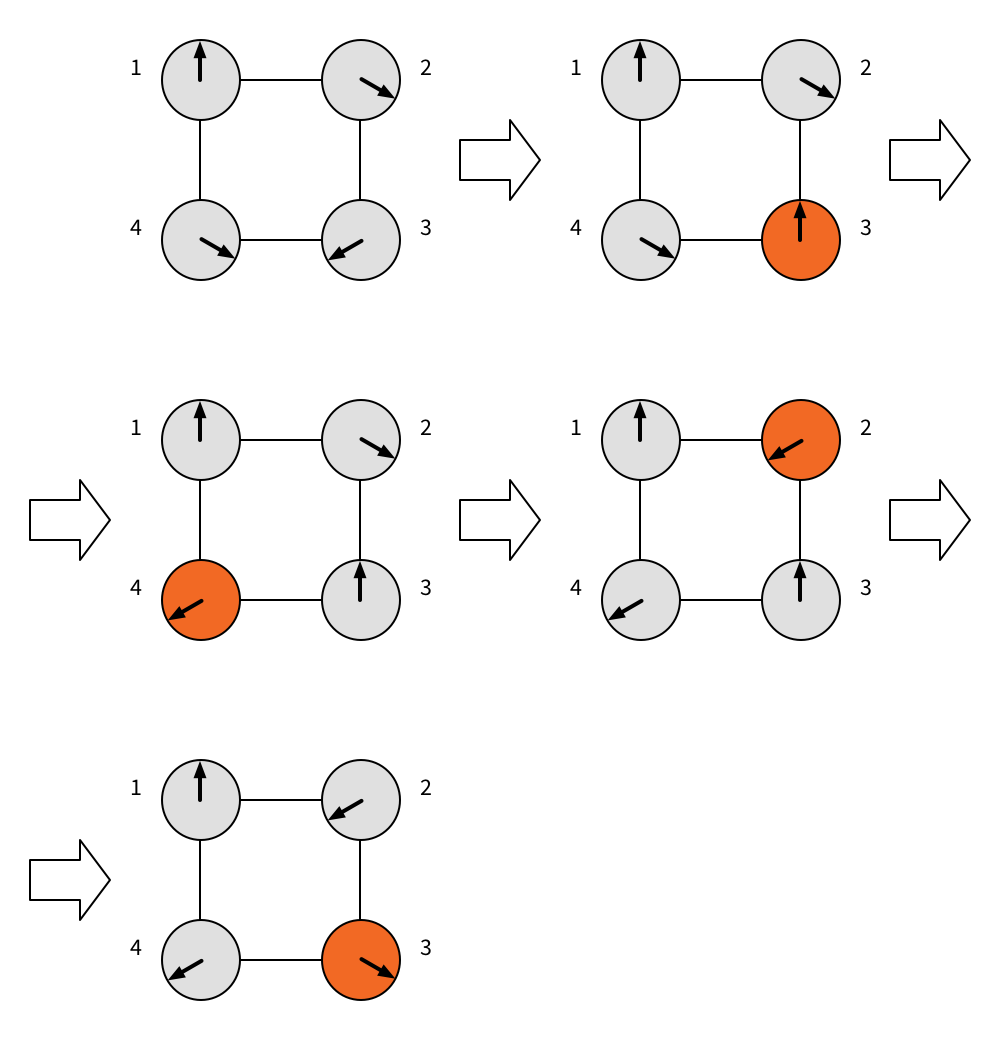Code Submission Evaluation System Login

HIIT Open 2018

 Start: 2018-05-26 11:00:00 End: 2018-05-26 16:00:00

CSES - HIIT Open 2018 - KnobsCSES - Knobs

Knobs

 Time limit: 2.00 s Memory limit: 512 MB

You have a graph with $n$ nodes and $m$ edges. Each node has a knob that you can turn clockwise or counterclockwise. Each knob can be in one of three positions: 0°, 120°, 240°. Initially, knob $i$ is in position $a_i$.

For each knob $i$ you also know its final position, $b_i$. You will need to find a way to turn knobs so that:
• in each step you turn only one knob (from one position to another position)
• two knobs that are connected by an edge are never in the same position
• after you are done, each knob is in its final position
Note that you can turn the same knob many times, to any of the positions.

Input

The first input line has two integers $n$ and $m$: the number of knobs and edges.

Then there are $n$ lines, each with two numbers $a_i$ and $b_i$. These indicate the current position $a_i$ and the final position $b_i$ of knob $i$.

Finally, there are $m$ lines, each with two numbers $i$ and $j$. This indicates that there is an edge between nodes $i$ and $j$; here $1 \le i < j \le n$.

The input configuration and the final configuration are valid: if there is an edge between knobs $i$ and $j$, then $a_i \ne a_j$ and $b_i \ne b_j$.

All inputs are such that if a solution exists, there is at least one solution with at most $10^5$ steps.

Output

If there is a way to turn knobs so that you can reach the final configuration, print a sequence that shows how to do it. First print one line with a number $s$ (at most $5 \cdot 10^5$): the number of steps. Then print $s$ lines, each with a pair of numbers, $x_k$ and $p_k$: in step $k$ you turn knob $x_k$ to position $p_k$. Here $1 \le x_k \le n$ and $p_k$ is 0, 120, or 240.

If there is no way to solve the problem, print only one line with value $-1$.

Constraints
• $1 \le n \le 20000$
• $1 \le m \le 20000$
Example

Input:
4 4 0 0 120 240 240 120 120 240 1 2 2 3 3 4 1 4

Output:
4 3 0 4 240 2 240 3 120

The following illustration shows the solution with four steps. Here 0° points up, 120° down right and 240° down left.